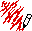## The Problem

Here's the definition of `intersect` given by Graham in Chapter 9, extended to show what it would like if cubes were added:

```(defun intersect (s pt xr yr zr)
(funcall (typecase s
(sphere #'sphere-intersect)
(cube #'cube-intersect))
s pt xr yr zr))```

As noted, this approach means that we will have to redefine `intersect` every time we define a new kind of object . Furthermore, whereever `intersect` should go, it definitely doesn't belong in the spheres definition file, because of the reference to cubes. On the other hand, we'd rather not put it in the base file where `tracer` and so on are defined because

• it would make the base file dependent on every object definition file
• we'd have to edit the base file and recompile it every time we added a new kind of object
• we'll get "undefined function" errors when `intersect` is compiled if any of the object files are missing, even if we don't need them

## The Data-Driven Solution

The data-driven approach would define `intersect` in the base file as follows:

```(defun intersect (s pt xr yr zr)
(let ((intersecter (get-intersecter s)))
(cond ((null intersecter)
(error "Unknown object type: ~S" s))
(t (funcall intersecter s pt xr yr zr)))))```

This code makes no explicit reference to any kind of object. Instead it calls get-intersecter to look up the intersect function for an object in a table of some kind. We can define `get-intersecter` in the base file this way:

```(defvar *intersecters* (make-hash-table))

(defun get-intersecter (s)
(gethash s *intersecters*))

(defun (setf get-intersecter) (fn s)
(setf (gethash s *intersecters*) fn))```

Or, if we use the tables package, we could simply say:

`(deftable get-intersecter)`

In any case, our definitions of `intersect` and `get-intersect` are object-independent. The data, stored in the table, drives what `intersect` does.

In the spheres definition file, we put the following:

`(setf (get-intersecter 'sphere) #'sphere-intersect)`

In the cubes definition file, we put

`(setf (get-intersecter 'cube) #'cube-intersect)`

Each definition file, in other words, takes care of putting the appropriate ray intersection function into the table. It's easy to add new objects, or to remove them, without editing or recompiling the base code.

Comments?Send mail to Chris Riesbeck.